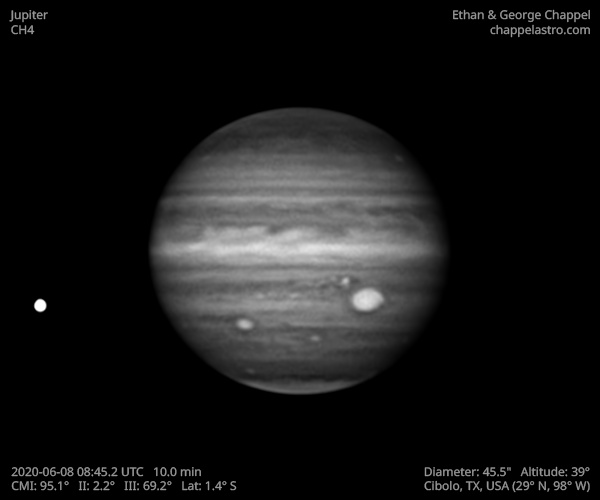# Jupiter 2020-06-08 08:45 UTC

CM1: 95.10°

CM2: 2.20°

CM3: 69.20°

CLat: -1.40°

Description

Variable conditions this morning, but enough data was recorded to make a good image.

Notes:

• The NEB has a few rifts past the central meridian.
• The Great Red Spot has a few visible features to the north of its dark core.
• Clyde's Spot, southwest of the GRS, is turning dark.
• Oval BA is rising.

Equipment

ZWO ASI290MM

Celestron EdgeHD 14

Logs
```FireCapture v2.6  Settings
------------------------------------
Observer=Ethan Chappel
Location=Cibolo
Scope=Celestron C14 EdgeHD
Camera=ZWO ASI290MM
Filter=CH4
Profile=Jupiter
Diameter=45.43"
Magnitude=-2.61
CMI=96.4° CMII=3.4° CMIII=70.5°  (during mid of capture)
FocalLength=3550mm (F/9)
Resolution=0.17"
Filename=2020-06-08-0847_7-EC-CH4-Jup.ser
Date=2020_06_08
Start=08_45_17.354
Mid=08_47_47.369
End=08_50_17.384
Start(UT)=08_45_17.354
Mid(UT)=08_47_47.369
End(UT)=08_50_17.384
Duration=300.030s
Date_format=yyyy_MM_dd
Time_format=HH_mm_ss
LT=UT -6h
Frames captured=5001
File type=SER
Binning=no
ROI=576x362
ROI(Offset)=0x0
FPS (avg.)=16
Shutter=60.00ms
Gain=450 (75%)
AutoGain=off
SoftwareGain=10 (off)
FPS=100 (off)
HighSpeed=on
USBTraffic=50 (off)
AutoHisto=75 (off)
Gamma=50 (off)
Brightness=1 (off)
AutoExposure=off
Histogramm(min)=0
Histogramm(max)=163
Histogramm=63%
Noise(avg.deviation)=4.06
Limit=300 Seconds
Sensor temperature=32.6°C
Focuser position=760
FireCapture v2.6  Settings
------------------------------------
Observer=Ethan Chappel
Location=Cibolo
Scope=Celestron C14 EdgeHD
Camera=ZWO ASI290MM
Filter=CH4
Profile=Jupiter
Diameter=45.43"
Magnitude=-2.61
CMI=93.3° CMII=0.4° CMIII=67.4°  (during mid of capture)
FocalLength=3550mm (F/9)
Resolution=0.17"
Filename=2020-06-08-0842_7-EC-CH4-Jup.ser
Date=2020_06_08
Start=08_40_13.710
Mid=08_42_43.734
End=08_45_13.758
Start(UT)=08_40_13.710
Mid(UT)=08_42_43.734
End(UT)=08_45_13.758
Duration=300.048s
Date_format=yyyy_MM_dd
Time_format=HH_mm_ss
LT=UT -6h
Frames captured=4979
File type=SER
Binning=no
ROI=576x362
ROI(Offset)=0x0
FPS (avg.)=16
Shutter=60.00ms
Gain=450 (75%)
AutoGain=off
SoftwareGain=10 (off)
FPS=100 (off)
HighSpeed=on
USBTraffic=50 (off)
AutoHisto=75 (off)
Gamma=50 (off)
Brightness=1 (off)
AutoExposure=off
Histogramm(min)=0
Histogramm(max)=154
Histogramm=60%
Noise(avg.deviation)=4.06
Limit=300 Seconds
Sensor temperature=32.8°C
Focuser position=760
```